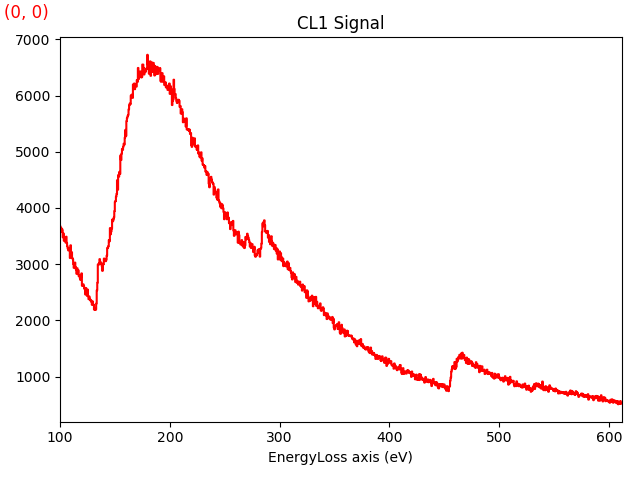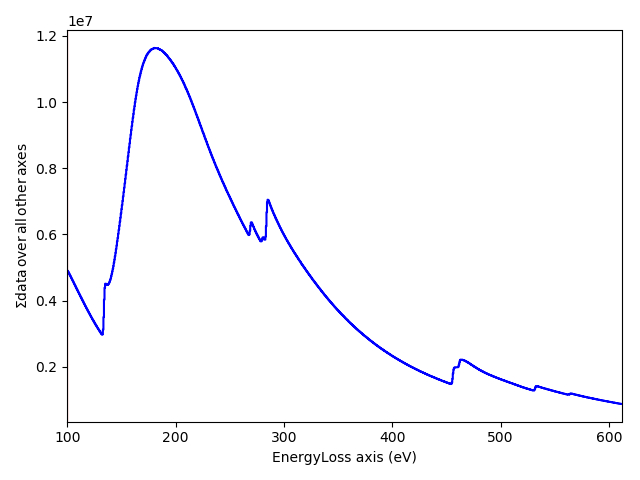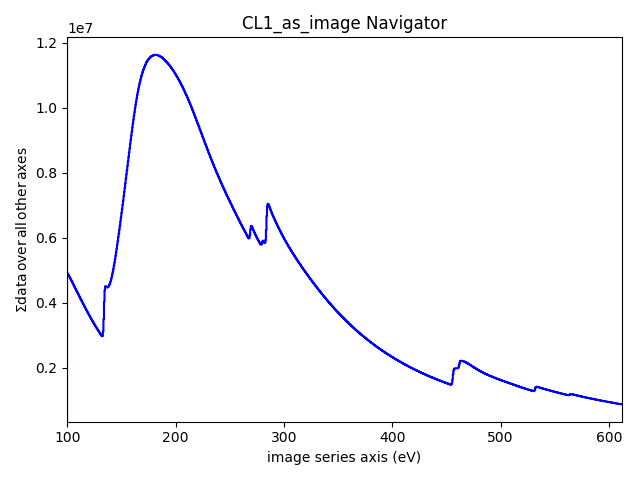# Getting started¶

Requires HyperSpy 1.3 or above

## Summary¶

This tutorial shows how to load, save and visualise data with HyperSpy as well as other basic functionalities.

Although not strictly required, some knowledge of Python can help with getting the most out of HyperSpy. If you are new to Python, the official tutorial is an excellent way to start.

This tutorial can be enjoyed interactively thanks to the Jupyter Notebook and IPython. If you are not familiar with the Jupyter Notebook, having a look at the Help menu above and the IPython documentation is highly recommended.

To save this webpage as an interactively useable IPython notebook, click on the "download" icon at the top-right of the webpage and save the resulting code with the suffix ".ipynb" (this should be proposed by default). If Hyperspy has been installed, the notebook can then be launched by double-clicking the saved file (Mac and Linux) or right-clicking the folder containing the file (Windows) and then clicking again on the notebook filename in the tab that will have opened in your browser. This will open another tab containing the interactive version of this page.

Important note: in the Jupyter notebook, to execute a command or group of commands in a cell, place the cursor in the cell and press 'shift+return'.

### Credits and changes¶

• 22/8/2016 Michael Walls. Include some more comments and explanations
• 9/8/2016 Francisco de la Peña. Update it for HyperSpy 1.1
• 27/7/2016 Francisco de la Peña. Update it for HyperSpy 1.0.1.
• 6/3/2016 Francisco de la Peña. Adapted from previous tutorials for the SCANDEM workshop.

IMPORTANT: Before you start create/download the datasets executing the code in the Appendix.

## 1. Importing HyperSpy¶

As any other Python library, to use HyperSpy first need to "import" it. The public HyperSpy API can be imported executing

import hyperspy.api as hs



However, in order to enable interactive plotting in IPython we need to activate the matplotlib "backend" first using the %matplotlib IPython magic.

NOTE: A "backend" in this context refers to the code determining way in which plotted data will be displayed. In the online version of this document we use the inline backend that displays non-interactive figures inside the Jupyter Notebook. However, for interactive data analysis purposes most would prefer to use the qt4, wx or nbagg backends.

In :
# This is a Python comment line - anything after a hashtag is a non-executed comment
%matplotlib nbagg
# You can replace 'nbagg' with any other available toolkit e.g 'qt4', 'tk'...
import hyperspy.api as hs
# Don't worry about the warning below, they're just for information

WARNING:hyperspy_gui_traitsui:The nbAgg matplotlib backend is not supported by the installed traitsui version and the ETS toolkit has been set to null. To set the ETS toolkit independently from the matplotlib backend, set it before importing matplotlib.
WARNING:hyperspy_gui_traitsui:The traitsui GUI elements are not available.


## 2. Getting help¶

HyperSpy documentation includes

• The Use Guide
• The docstrings (see below)
• The demos such as this one.

### Docstrings¶

In Python most objects include their own documentation (docstring in Python jargon). In the Jupyter notebook you can consult the documentation interactively by:

• If the object is a function or a method, by pressing the Shift + Tab keys after writing the first brackets, e.g. load(<Shift + Tab>

All HyperSpy public objects are contained in the hs variable that we have imported above. Let's practice the different methods to access the docstrings by inspecting the hs doctring:

In :
hs?


The dir function is very helpful to inspect the attributes of Python objects

In :
dir(hs)

Out:
['__builtins__',
'__cached__',
'__doc__',
'__file__',
'__name__',
'__package__',
'__spec__',
'_logger',
'datasets',
'eds',
'get_configuration_directory_path',
'hyperspy',
'hyperspy_gui_ipywidgets',
'hyperspy_gui_traitsui',
'interactive',
'logging',
'markers',
'material',
'model',
'model_selection',
'plot',
'preferences',
'roi',
'samfire',
'set_log_level',
'signals',
'stack',
'transpose']

## 3. Structure overview¶

HyperSpy provides (among other things):

• A collection of "signals" which are specialised data containers with functions (methods in Python jargon) that operate on the data. They can be found in hs.signals.
• Functions that operate on the signals. For example hs.stack to stack signals and the several functions in hs.plot.
• A collection of "model" classes that generate models (usually for fitting) by linearly combining the components in hs.model.components.
• A database of chemical elements with EELS ionisation edges and X-ray lines in hs.material.
• Some example data in hs.datasets
In :
dir(hs.signals)

Out:
['BaseSignal',
'ComplexSignal',
'ComplexSignal1D',
'ComplexSignal2D',
'DielectricFunction',
'EDSSEMSpectrum',
'EDSTEMSpectrum',
'EELSSpectrum',
'HologramImage',
'Signal1D',
'Signal2D',
'__builtins__',
'__cached__',
'__doc__',
'__file__',
'__name__',
'__package__',
'__spec__']

To create a HyperSpy signal, just pass some data to one of the signals in hs.signals e.g.

In :
ten = hs.signals.Signal1D([1, 2, 3, 4, 5, 6, 7, 8, 9, 10])


Now the ten variable contains a Signal1D instance.

Note that, thanks to IPython, there is no need to type all the commands or paths manually—it is enough to write the first letters and press the Tab key.

In :
ten

Out:
<Signal1D, title: , dimensions: (|10)>

Most of the operations that we can performs in the data are available inside this object, and can be accessed by writing a dot i.e. . after the name of the variable, pressing the Tab key and choosing an option from the list that appears. Alternatively, use the dir function to print them all.

In :
dir(ten)

Out:
['T',
'__abs__',
'__and__',
'__array__',
'__array_wrap__',
'__call__',
'__class__',
'__deepcopy__',
'__delattr__',
'__dict__',
'__dir__',
'__divmod__',
'__doc__',
'__eq__',
'__floordiv__',
'__format__',
'__ge__',
'__getattribute__',
'__gt__',
'__hash__',
'__iand__',
'__ifloordiv__',
'__ilshift__',
'__imod__',
'__imul__',
'__init__',
'__init_subclass__',
'__invert__',
'__ior__',
'__ipow__',
'__irshift__',
'__isub__',
'__iter__',
'__itruediv__',
'__ixor__',
'__le__',
'__len__',
'__lshift__',
'__lt__',
'__mod__',
'__module__',
'__mul__',
'__ne__',
'__neg__',
'__new__',
'__next__',
'__or__',
'__pos__',
'__pow__',
'__reduce__',
'__reduce_ex__',
'__repr__',
'__rshift__',
'__setattr__',
'__sizeof__',
'__str__',
'__sub__',
'__subclasshook__',
'__truediv__',
'__weakref__',
'__xor__',
'_alias_signal_types',
'_apply_function_on_data_and_remove_axis',
'_assign_subclass',
'_auto_reverse_bss_component',
'_binary_operator_ruler',
'_calculate_recmatrix',
'_calculate_summary_statistics',
'_change_API_comp_label',
'_check_signal_dimension_equals_one',
'_check_signal_dimension_equals_two',
'_data',
'_data_aligned_with_axes',
'_deepcopy_with_new_data',
'_dtype',
'_estimate_poissonian_noise_variance',
'_export_factors',
'_get_array_slices',
'_get_factors',
'_get_plot_title',
'_get_signal_signal',
'_get_undefined_axes_list',
'_integrate_in_range_commandline',
'_iterate_signal',
'_lazy',
'_ma_workaround',
'_make_sure_data_is_contiguous',
'_map_all',
'_map_iterate',
'_plot',
'_plot_factors_or_pchars',
'_plot_permanent_markers',
'_print_summary',
'_remove_axis',
'_remove_background_cli',
'_replot',
'_signal_dimension',
'_signal_type',
'_slicer',
'_spikes_diagnosis',
'_summary',
'_to_dictionary',
'_unary_operator_ruler',
'_unfold',
'_unmix_components',
'_validate_rebin_args_and_get_factors',
'align1D',
'as_lazy',
'as_signal1D',
'as_signal2D',
'axes_manager',
'blind_source_separation',
'calibrate',
'change_dtype',
'copy',
'create_model',
'crop',
'crop_signal1D',
'data',
'decomposition',
'deepcopy',
'derivative',
'diff',
'estimate_peak_width',
'estimate_poissonian_noise_variance',
'estimate_shift1D',
'events',
'export_bss_results',
'export_decomposition_results',
'filter_butterworth',
'find_peaks1D_ohaver',
'fold',
'gaussian_filter',
'get_bss_factors',
'get_bss_model',
'get_current_signal',
'get_decomposition_factors',
'get_decomposition_model',
'get_dimensions_from_data',
'get_explained_variance_ratio',
'get_histogram',
'hanning_taper',
'inav',
'indexmax',
'indexmin',
'integrate1D',
'integrate_in_range',
'integrate_simpson',
'interpolate_in_between',
'is_rgb',
'is_rgba',
'is_rgbx',
'isig',
'learning_results',
'map',
'max',
'mean',
'min',
'models',
'nanmax',
'nanmean',
'nanmin',
'nanstd',
'nansum',
'nanvar',
'normalize_bss_components',
'normalize_decomposition_components',
'normalize_poissonian_noise',
'plot',
'plot_bss_factors',
'plot_bss_results',
'plot_cumulative_explained_variance_ratio',
'plot_decomposition_factors',
'plot_decomposition_results',
'plot_explained_variance_ratio',
'print_summary_statistics',
'rebin',
'remove_background',
'reverse_bss_component',
'reverse_decomposition_component',
'rollaxis',
'save',
'set_signal_origin',
'set_signal_type',
'shift1D',
'smooth_lowess',
'smooth_savitzky_golay',
'smooth_tv',
'spikes_removal_tool',
'split',
'squeeze',
'std',
'sum',
'swap_axes',
'tmp_parameters',
'to_signal2D',
'transpose',
'undo_treatments',
'unfold',
'unfold_signal_space',
'unfolded',
'update_plot',
'valuemax',
'valuemin',
'var']

For example:

In :
ten.print_summary_statistics()

Summary statistics
------------------
mean:	5.500
std:	2.872

min:	1.000
Q1:	3.250
median:	5.500
Q3:	7.750
max:	10.000


### Very useful tip: "Autocompletion"¶

In fact, long commands like the previous one can be entered more quickly using the tab key. Just enter the first few letters of the command, press tab and a list of the possible commands will appear. Navigate to the required command with the arrow keys (you still need the brackets at the end). If you are in interactive mode, try it in the cell below:

In [ ]:
ten.p


More typically we load data from files using the hs.load function. The following code loads the CL1.rpl file in the machine_learning folder and stores it in the s variable.

In :
s = hs.load("machine_learning/CL1.hdf5")


Let's check what is inside the s variable

In :
s

Out:
<EELSSpectrum, title: , dimensions: (64, 64|1024)>

HyperSpy has loaded the data into an EELSSpectrum object that we have stored in the s variable. The symbol | separates the navigation dimensions x, y and the signal dimensions, in this case energy loss.

The metadata read from the file is stored in the original_metadata attribute.

In :
s.original_metadata

Out:
├── beam-energy = 100.0
├── byte-order = dont-care
├── collection-angle = 10.0
├── convergence-angle = 7.0
├── data-length = 8
├── data-type = float
├── depth = 1024
├── depth-name = EnergyLoss
├── depth-origin = 100.0
├── depth-scale = 0.5
├── depth-units = eV
├── ev-per-chan = 1.0
├── height = 64
├── height-name = Y
├── height-origin = 0.0
├── height-scale = 1.0
├── height-units = cm
├── key = value
├── offset = 0
├── record-by = vector
├── signal = EELS
├── width = 64
├── width-name = X
├── width-origin = 0.0
├── width-scale = 1.0
└── width-units = cm

Part of this information is also available in the metadata attribute. HyperSpy only uses internally the information in metadata.

In :
s.metadata

Out:
├── Acquisition_instrument
│   └── TEM
│       ├── Detector
│       │   └── EELS
│       │       └── collection_angle = 10.0
│       ├── beam_energy = 100.0
│       └── convergence_angle = 7.0
├── General
│   ├── original_filename = CL1.rpl
│   └── title =
└── Signal
├── binned = True
├── signal_origin =
└── signal_type = EELS

The metadata can be easily modified:

In :
s.metadata.Acquisition_instrument.TEM.convergence_angle = 10

In :
s.metadata

Out:
├── Acquisition_instrument
│   └── TEM
│       ├── Detector
│       │   └── EELS
│       │       └── collection_angle = 10.0
│       ├── beam_energy = 100.0
│       └── convergence_angle = 10
├── General
│   ├── original_filename = CL1.rpl
│   └── title =
└── Signal
├── binned = True
├── signal_origin =
└── signal_type = EELS

## 3. Axis properties¶

The axes are stored in the axes_manager attribute:

In :
s.axes_manager

Out:

< Axes manager, axes: (64, 64|1024) >

Navigation axis name size index offset scale units
X 64 0 0.0 1.0 cm
Y 64 0 0.0 1.0 cm
Signal axis name size offset scale units
EnergyLoss 1024 100.0 0.5 eV

The AxesManager can be indexed:

In :
s.axes_manager

Out:
<X axis, size: 64, index: 0>

It is also possible to access the axes by name:

In :
s.axes_manager["EnergyLoss"]

Out:
<EnergyLoss axis, size: 1024>

The axes have offset, scale, units and name attributes

In :
s.axes_manager["EnergyLoss"].scale

Out:
0.5
In :
s.axes_manager["EnergyLoss"].units

Out:
'eV'

## 4. Visualisation¶

In :
s.plot()### Moving around¶

• Using the keyboard arrow keys
• Using the pointer

### Other shortcuts¶

• Two pointers: enable/disable by pressing e
• Adjust image contrast: press h
• Increase/decrease the pointer size: + and - keys

When using HyperSpy, it is common to have many open figures at a given time. The close matplotlib command is useful to close all the images at once, but, for that, first we have to import matplotlib:

In :
import matplotlib.pyplot as plt
plt.close("all")


## 5. Signal and navigation axes¶

We can change the way in which Hyperspy "sees" the data by converting the EELSSpectrum into a Signal2D object

In :
im = s.to_signal2D()

WARNING:hyperspy.signal:<Signal2D, title: , dimensions: (1024|64, 64)> data is replaced by its optimized copy


The im variable now contains a Signal2D object that shares the data with the EELSSpectrum object in s.

In :
im

Out:
<Signal2D, title: , dimensions: (1024|64, 64)>

Now we can visualize the same data in the "energy filtered" way

In :
im.plot()## 6. Saving to file¶

In :
im.save('CL1_as_image', overwrite=True)


By default HyperSpy writes to the HDF5 file format.

To save to another format you must specify the extension, e.g.:

In :
im.save('CL1_as_image.tif', overwrite=True)


We can load it to verify that we do get back what we saved

In :
im = hs.load('CL1_as_image.tif')

In :
im.plot()## 7. Indexing¶

HyperSpy signals can be indexed using the isig and inav attributes. Indexing is a very powerful feature. To go beyond the basic examples here have a look at the User Guide.

Firstly we'll load an RGB image

In :
im = hs.load("astronaut.hdf5")


Notice that the navigation dimension is 3 because there is one axis per colour channel.

In :
im

Out:
<Signal2D, title: , dimensions: (3|512, 512)>

Let's plot the three channels:

In :
hs.plot.plot_images(im, axes_decor="off", colorbar=False, label=["R", "G", "B"])Out:
[<matplotlib.axes._subplots.AxesSubplot at 0x7f5b49ec9630>,
<matplotlib.axes._subplots.AxesSubplot at 0x7f5aed2e1f60>,
<matplotlib.axes._subplots.AxesSubplot at 0x7f5aed269dd8>]

We can index the navigation axes using inav. For examples to obtain just the image in the first channel (R):

In :
im.inav.plot()And for the last two channels (G, B)

In :
hs.plot.plot_images(im.inav[1:], axes_decor="off", colorbar=False)